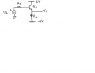# Need help biasing this transistor circuit

• FOIWATER

#### FOIWATER

Gold Member
I am having troubles biasing this transistor circuit

Mainly, I cannot find Ic

The only equation I can come up w/ is 6 - Vce - ie*Re - (-6) = 0

but it doesn't help me, as I do not Vce

I do not know how to find Ib, Vs is messing me up, I am not sure how to analyze this...

The given values are beta 100 Vbe sat 0.3 Vbe 0.7 Va = 100 (early voltage)

this isn't homework, btw

#### Attachments

•ppppp.png
4.4 KB · Views: 501
Last edited:
My only thought would be to midpoint bias it? idk,
say that at cutoff Vce = Vcc - Vee = 12 volts, and take half as 6 volts for Vce (bias)
Ic (sat) approx = Ie sat ------> 12 - Vcesat (0.3) - Re * ic = 0
Given Re I could solve for Ic, then take half?

I see an emitter follower where the emitter voltage will be roughly 0.7V less than Vs minus the drop across Rs.

Ic will be determined by the voltage across Rl with [Vs -(drop across Rs) - Vbe] on one side and -6V on the other.

Drop across Rs is Ic/Beta. Vbe is around 0.7V.

Do you understand how the emitter follower works? Basically the transistor supplies whatever current is required for the emitter voltage to be a Vbe drop (about 0.7V) less than the base voltage.

yes I understand that, I do not know Vs value, though

So I am still unsure how to find what that current would be

If you don't know Vs you can't determine anything. So I am confused about what you are trying to determine.

Basically Ic = (Vs - (-6))/Rl not accounting for Vbe or drop across Rs.

Yeah I would agree with all those things.

It's just that I am doing a course in analog ccts soon, and this was a question on an old exam from another teacher.

Vs was not given

Then you write the equation in Vs like I did. The answer has to have the variable Vs in it and I've given you all the pieces.

Why for most biasing circuits is a signal Vs not present then?

for example a voltage divider biasing cct, or a base biasing cct?

There is not usually an ac input

Vs isn't part of the biasing arrangement, it represents the signal to be buffered/amplified. Most similar circuits, to be useful, allow a signal input! In many cases the signal is capacitor-coupled to the base, so it certainly is there. Whether the AC is shown as being in series with the DC, or in parallel with it, is immaterial, all that matters is that the signal be impressed upon the bias.

It might seem that there is no biasing voltage source in your circuit here; but there is, and it is zero volts. If the transistor base were connected by a piece of wire directly to ground it would mean the AC coupled to the base would also get shorted to ground, so not be amplified.

Q: what does "Va = 100 (early voltage)" mean?

So the signal here is impressed upon the bias? I don't understand what that means, although I've read it everywhere, I don't understand the meaning of it. Does it simply mean that, the bias point is calculated as if no signal is applied, then the ac signal is put on top of it? If so, how does this bias prevent noise at the output?

Va = early voltage, since the width of the base changes for changes in Vcb, the transistor has an additional resistance due to the early effect, the resistance is labelled ro and is calculated as Va (early voltage) divided by Vt (thermal voltage). It is usually quite high and can be ignored (in parallel with Rc in small signal model) but here there is no Rc, I am not sure even if this resistance goes in parallel with gmVbe, or in parallel with the load resistor, or where it does go)

So why again should the biasing voltage be zero volts? is it a rule to short the ac supply?

So the signal here is impressed upon the bias? I don't understand what that means, although I've read it everywhere, I don't understand the meaning of it. Does it simply mean that, the bias point is calculated as if no signal is applied, then the ac signal is put on top of it? If so, how does this bias prevent noise at the output?
The signal AC has zero average value, so the signal contributes nothing to the bias. The bias contributes no noise because we use a DC power supply which is smooth and well filtered.

Vs is not a biasing supply; the ~ indicates it is AC, so that source represents the input signal.

Thanks friend

With all the information given you should be able to easily determine the Ic for any given Vs value. This is not about "bias".

I propose you solve the following and post the answers:
Assume Beta = 100, Rs = 10K and Rl = 1K.

1. Calculate Ic for Vs = 0V DC.

2. Calculate Ic for Vs = +1 DC and Vs = -1v DC.

There is no bias as such. At least not in the sense that I think of when biasing a transistor circuit. It is a DC coupled emitter follower and the output voltage (at the emitter) follows the input voltage Vs. (offset by a few voltage drops which we have explained)